# How does flow rate affect the individual heat-transfer coefficients on the tube and annular sides...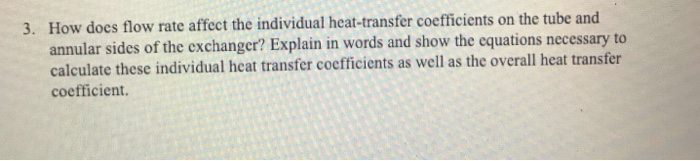How does flow rate affect the individual heat-transfer coefficients on the tube and annular sides of the exchanger? Explain in words and show the equations necessary to calculate these individual heat transfer coefficients as well as the overall heat transfer coefficient. 3.

The local heat transfer coefficient increases as the mass flow rate increases. The heat flow from the pipe wall in a length dx isAfter the integration from limits T1 (temperature at inlet x=0) to T in a length 0 to xThe temperature at the exit of the heat exchanger is T= T2 at x=LThe overall heat transfer coefficient is constant throughout the heat exchanger as per the LMTD assumption.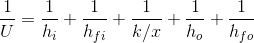##### Add Answer of: How does flow rate affect the individual heat-transfer coefficients on the tube and annular sides...
Similar Homework Help Questions
• ### HEAT AND MASS TRANSFER (HEAT EXCHANGERS)

A shell-and-tube heat exchanger (one shell pass, two tube passes) transfers heat from the hot exhaust gases of a furnace to a cold water stream. The exhaust gases,which have effectively the same properties as air, enter the device at 473 K and leave at 366 K. The water enters at 308 K and leaves at 358 K at a flow rate of 150kg/min. The overall heat transfer coefficient is 180 W/m2-K. Using the effectiveness-NTU method, calculate the area of the...

• ### Twenty [kw] of heat is to be removed from 375 [k] water flowing at 0.15 [kg/s] into the inner pipe of concentric tube heat exchanger. Cooling water enters the annulus at 290 [k] and leaves at 320...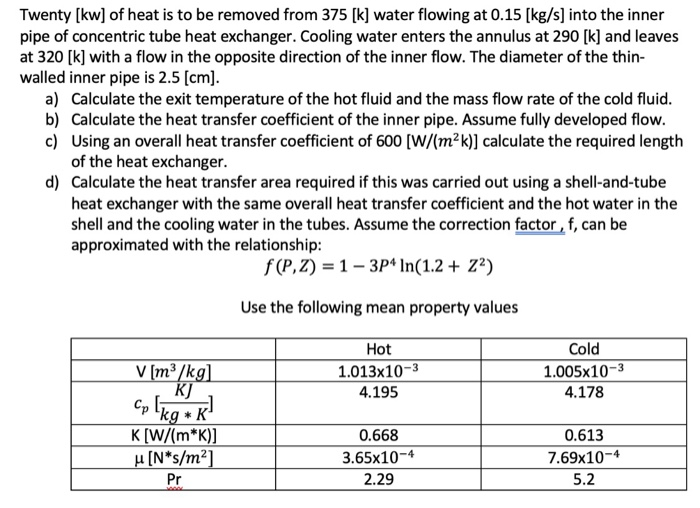Twenty [kw] of heat is to be removed from 375 [k] water flowing at 0.15 [kg/s] into the inner pipe of concentric tube heat exchanger. Cooling water enters the annulus at 290 [k] and leaves at 320 [k] with a flow in the opposite direction of the inner flow. The diameter of the thin- walled inner pipe is 2.5 [cm] a) b) c) Calculate the exit temperature of the hot fluid and the mass flow rate of the cold fluid...

• ### heat transfer question

3.) The mass flow rate, specific heat, and inlet temperature of the tube-side stream in a double-pipe, parallel-flow heat exchanger are 3200 kg/h, 2.0 kJ/kg·K, and120°C , respectively. The mass flow rate, specific heat, and inlet temperature of the other stream are 2000 kg/h, 4.2 kJ/kg·K, and 20°C, respectively. The heattransfer area and overall heat transfer coefficient are 0.5 m2 and 2.0 kW/m2·K, respectively. Find the outlet temperatures of both streams in steady operation usingthe (a) LMTD method and (b)...

• ### QUESTION 1: Heat Exchanges A concentric tube parallel floween s hot water saturated liquid from 30...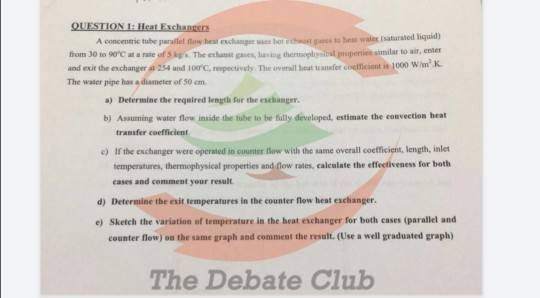QUESTION 1: Heat Exchanges A concentric tube parallel floween s hot water saturated liquid from 30 to 90%Catate of St. The exh , having thermopla properties similar to air, enter and exit the exchange 254 and 100 p ectively. The heat force 1000 W/mK The water pipe has a diameter of 50 cm a) Determine the required length for the exchanger. b) Assuming water flow inside the tube to be fully developed, estimate the convection heat transfer coefficient c) If...

• ### Heat transfer question

<ol><li>Assume a safety related counterflow heat exchanger that has a single phase liquid on both the shell and tube sides, and a constant specific heat for eachliquid.&#160; Under which of the following conditions does the heat transfer between the two liquids decrease measurably?</li></ol><ol><li>The flow rate of the hotter liquid is increased.</li><li>The flow rate of the cooler liquid is decreased.</li><li>The temperature of each liquid is increased.</li><li>The temperature of each liquid is decreased.</li></ol>

• ### QUESTION 4 (25 marks) Water (Cr 4.208 kJ/kg.K) at flow rate of 5.11 kg/s s heated from 78°C to 98°C in an economize...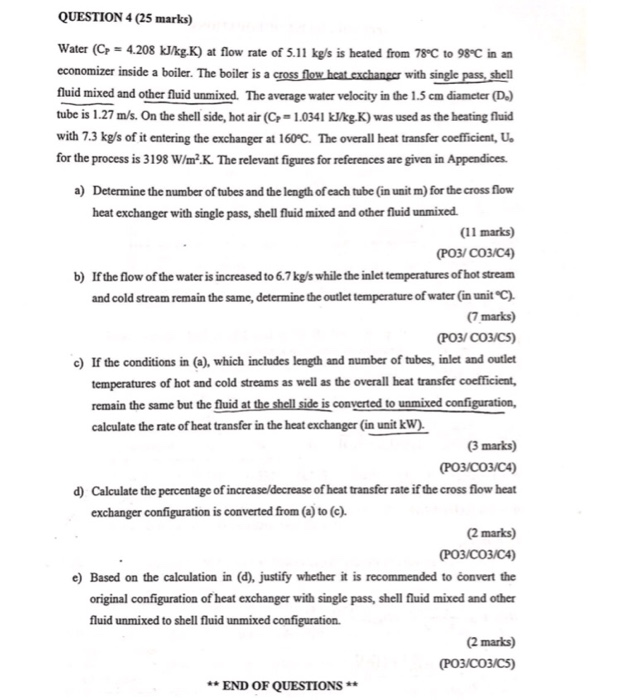QUESTION 4 (25 marks) Water (Cr 4.208 kJ/kg.K) at flow rate of 5.11 kg/s s heated from 78°C to 98°C in an economizer inside a boiler. The boiler is a cross flow heat exchanger with single pass, shell fluid mixed and other fluid unmixed. The average water velocity in the 1.5 cm diameter (D) tube is 1.27 m/s. On the shell side, hot air (C,-1.0341 kJ/kgK) was used as the heating fluid with 7.3 kg/s of it entering the exchanger...

• ### Water enters a tube at 27 C with a flow rate of 450 kg/h. The heat transfer from the tube wall...

Water enters a tube at 27 C with a flow rate of 450 kg/h. The heat transfer from the tube wall tothe fluid is given as qs (W/m) = ax, where the coefficient a = 20 W/m2 and x (m) is the actualdistance from the tube entrance.

• ### 3. The annular pipe arrangement shown in the figure below is made of stainless steel and the oute...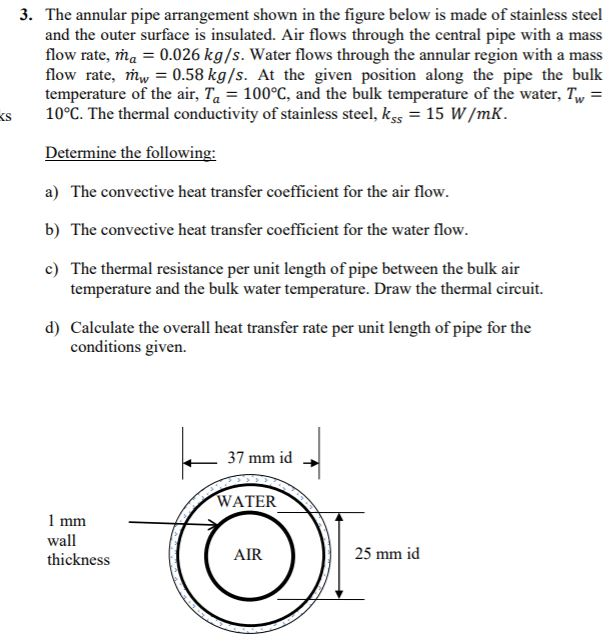3. The annular pipe arrangement shown in the figure below is made of stainless steel and the outer surface is insulated. Air flows through the central pipe with a mass flow rate, ma0.026 kg/s. Water flows through the annular region with a mass flow rate, mw-0.58 kg/s. At the given position along the pipe the bulk temperature of the air, Ta-100°C, and the bulk temperature of the water, Tw- s 10°C. The thermal conductivity of stainless steel, kss 15 W/mK....

• ### Please provide a step-by-step solution to this problem. Heat Transfer 1. The material should be f...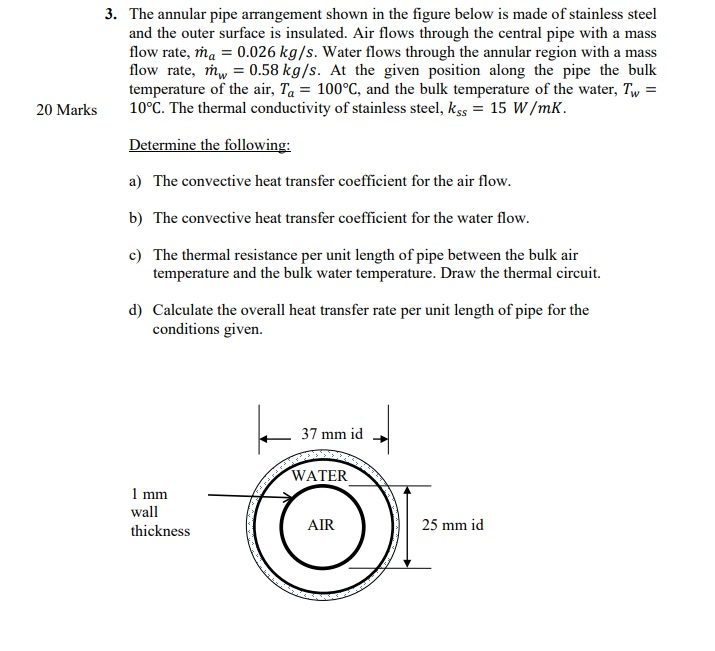Please provide a step-by-step solution to this problem. Heat Transfer 1. The material should be from the textbook (Fundamentals of Heat and Mass Transfer) or similar 3. The annular pipe arrangement shown in the figure below is made of stainless steel and the outer surface is insulated. Air flows through the central pipe with a mass flow rate, ma0.026 kg/s. Water flows through the annular region with a mass flow rate, mw 0.58 kg/s. At the given position along the...

• ### (b) Exhaust gases flowing through a tubular heat exchanger at the rate of 0.3 kg/s are cooled from 400 to 120°C by w...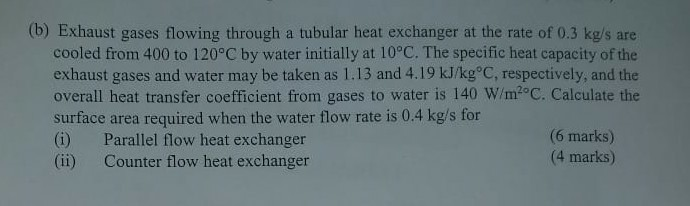(b) Exhaust gases flowing through a tubular heat exchanger at the rate of 0.3 kg/s are cooled from 400 to 120°C by water initially at 10°C. The specific heat capacity of the exhaust gases and water may be taken as 1.13 and 4.19 kJ/kg°C, respectively, and the overall heat transfer coefficient from gases to water is 140 W/m2°C. Calculate the surface area required when the water flow rate is 0.4 kg/s for (6 marks) (4 marks) (i) (ii) Parallel flow...

Need Online Homework Help?